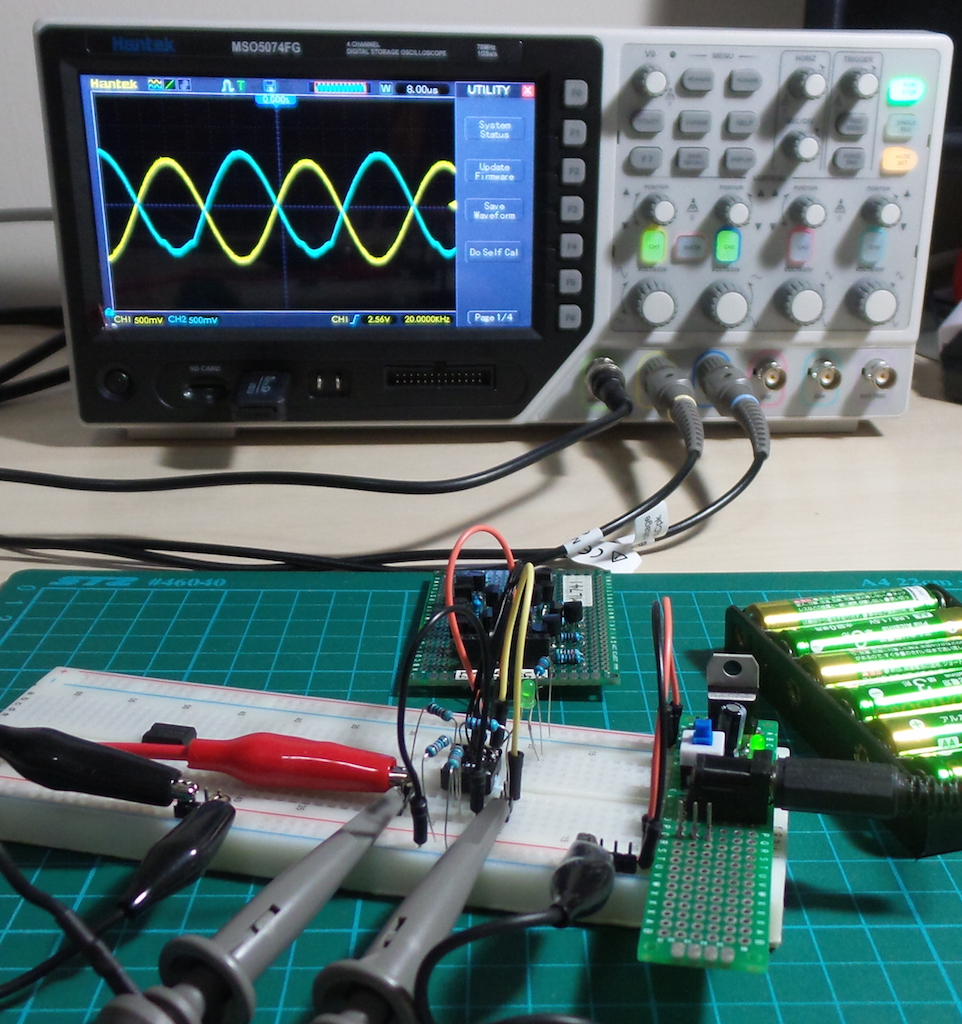# #158 ML741/Inverter

Test an inverter circuit using the ML741 discrete component opamp

This is a demonstration of an inverter/inverting buffer circuit using the ML741 discrete component opamp. The general operation of the circuit is for the output to follow the inverse of the inverting input. This is a special case of the general inverting amplifier configuration with gain set to -1, i.e.

``````Vout = -Vin
Rf = Rin
``````### How it works

Fundamentally, an op-amp strives to keep its inverting an non-inverting inputs equal by modulating the output.

In the inverter/inverting amplifier configuration, the inverting input is at the midpoint of the Rin:Rf voltage divider. Hence the op amp achieves inverting/non-inverting input equilibrium when Vout = -Vin.

## Construction

In this circuit, I am using a single rail supply (V- = GND) instead of the “conventional” dual rail supply (V+/V-).

For this reason, the non-inverting input is pegged to V+/2 with a voltage divider. In a dual rail configuration, V+/2 is usually “ground”.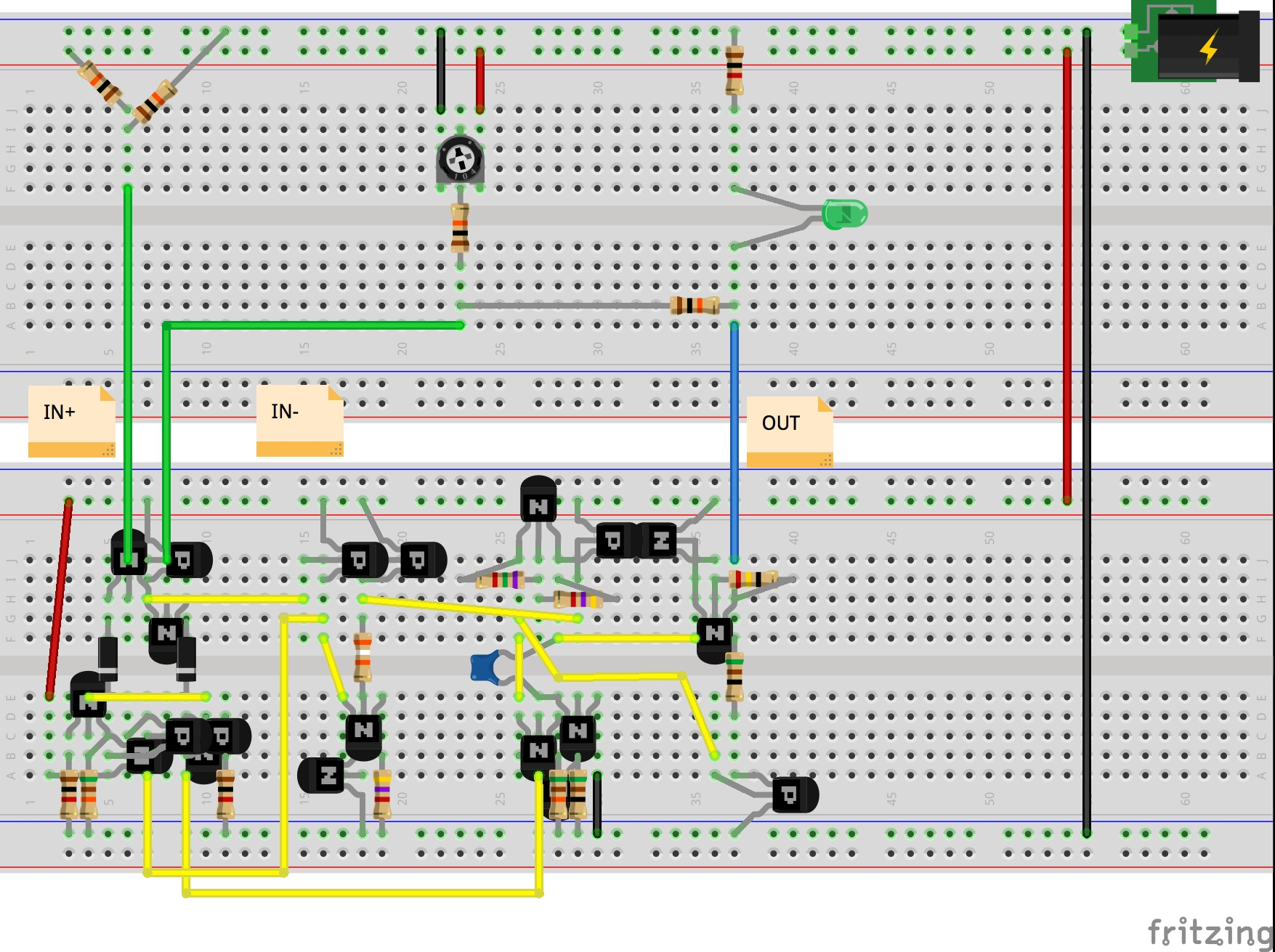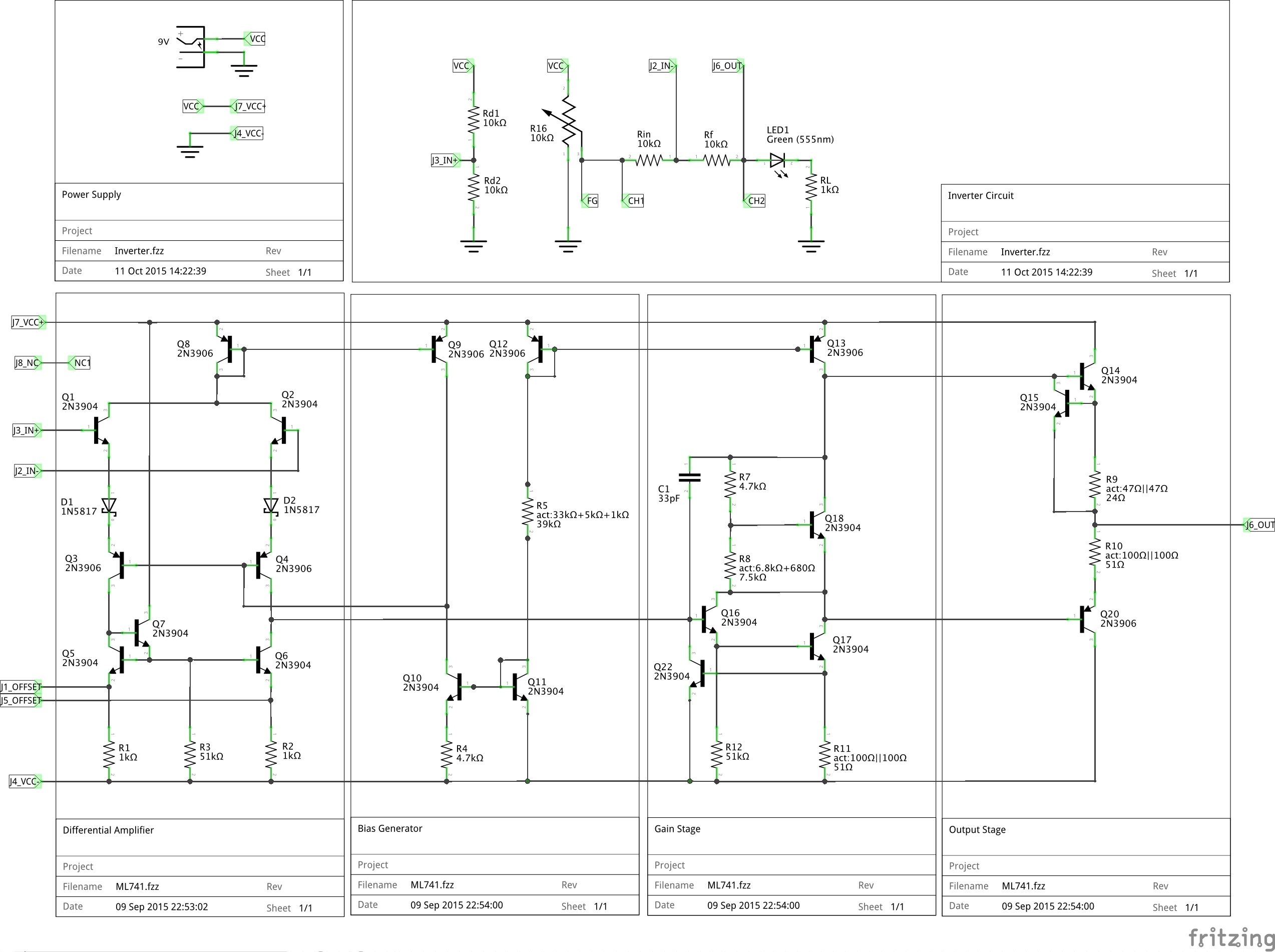ML741 on the right, and a standard UA741CN on the left waiting to be put to the test..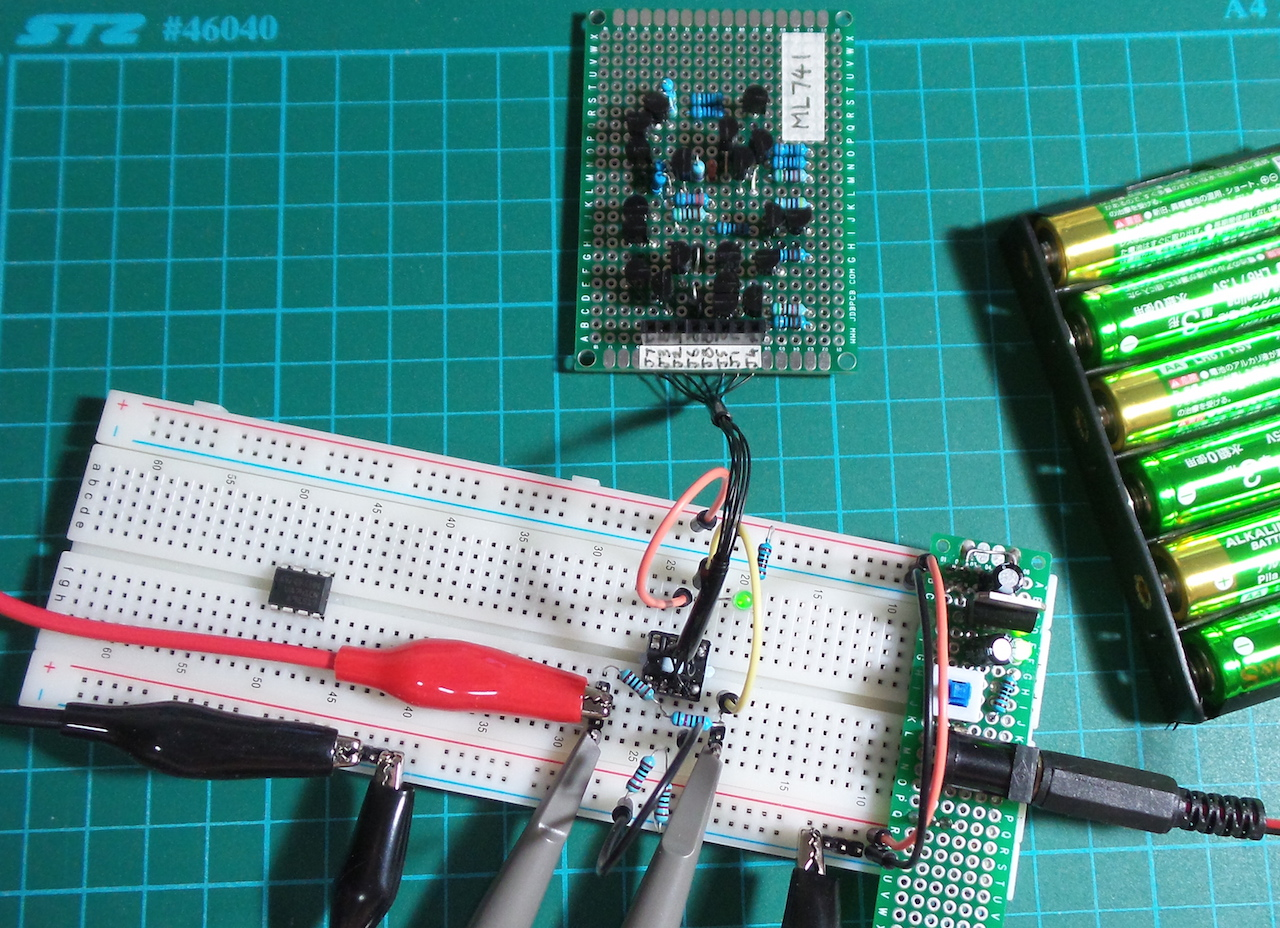## ML741 v “real” 741 Test

Here are some results comparing the behaviour of a standard UA741CN chip with the ML741 (protoboard version).

Setup:

• power is 5V single rail, i.e. V- = GND
• non-inverting input is fed a sine wave 1Vpp with 2.5V DC offset
• the function generator sine wave input replaces the manual 10kΩ input pot in the schematic above (at node FG)

Scope connections

• CH1: non-inverting input
• CH2: output

I’ve purposely driven the input signal such that it clips the lower output rail of both the ML741 and UA741CN, because things get interesting around the rails.

### At 1kHz

• ML741 is performing nicely
• UA741CN hitting the lower rail at ~1.9v

ML741: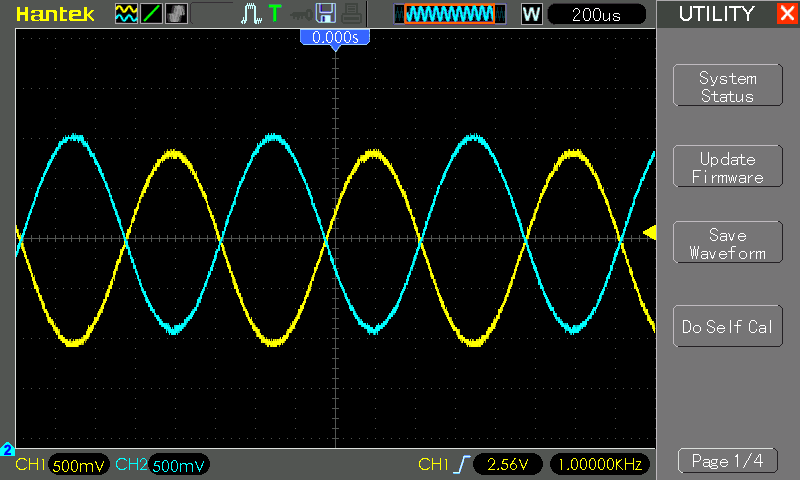UA741CN: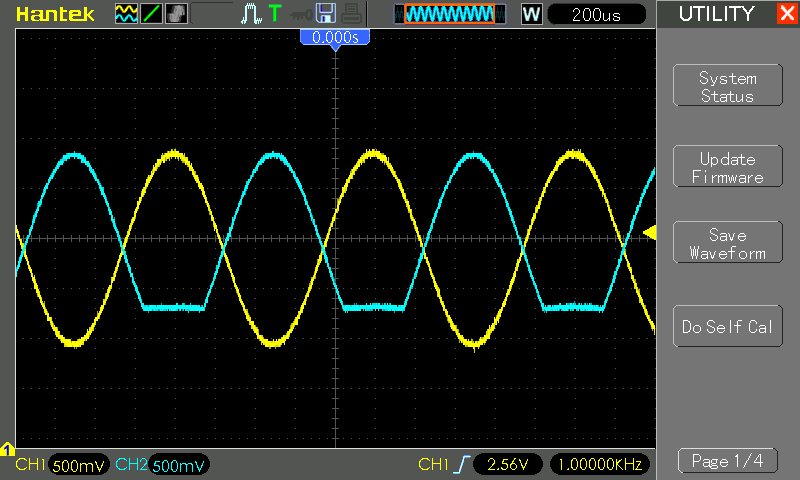### At 40kHz

• ML741 starting to distort at the lower end
• UA741CN still performing similar to 1kHz

ML741: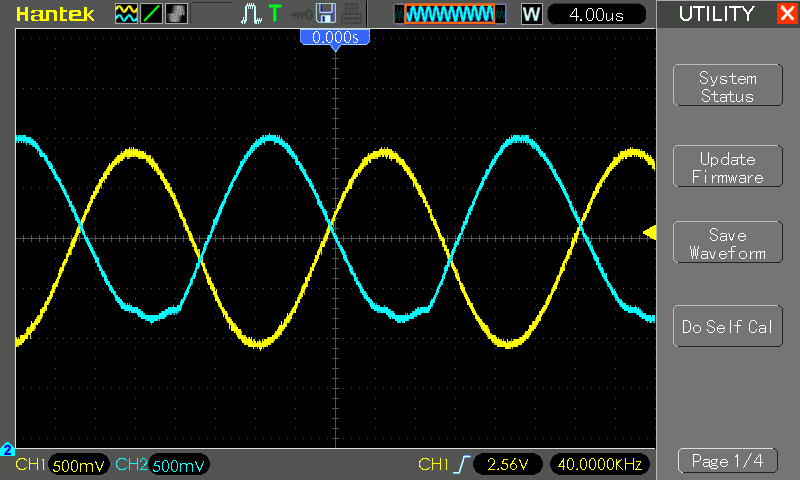UA741CN: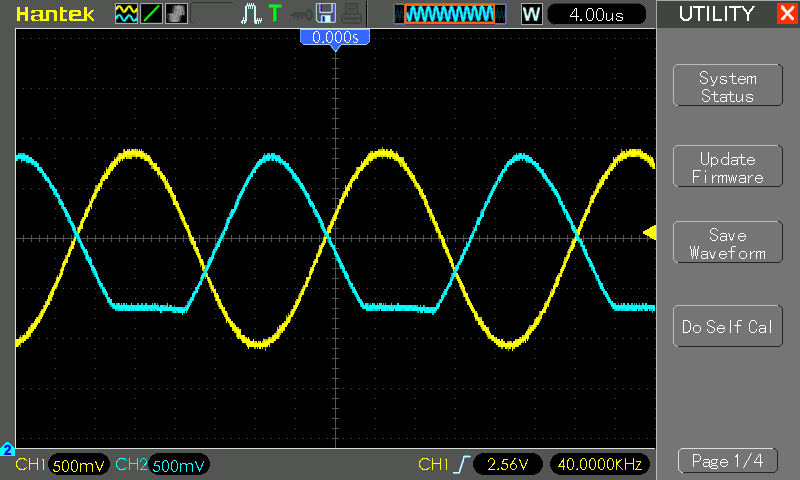### At 100kHz

Both ML741 and UA741CN exhibiting similar distortion patterns: waveforms have lost definition, are phase-shifted and attenuated

ML741: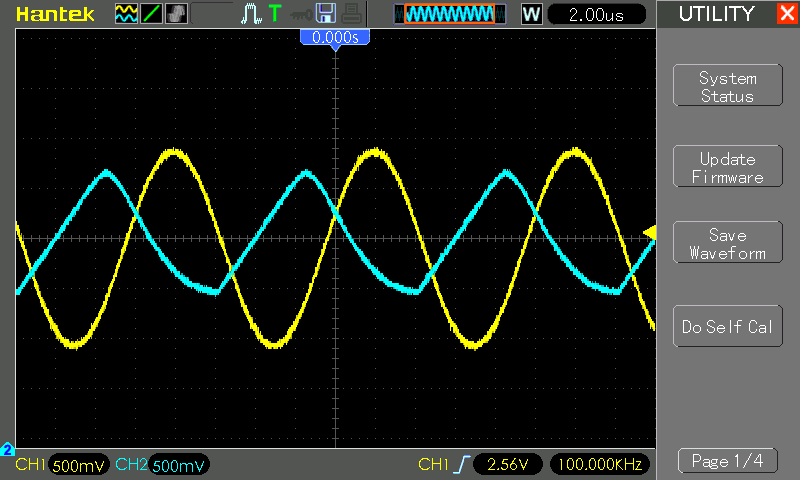UA741CN: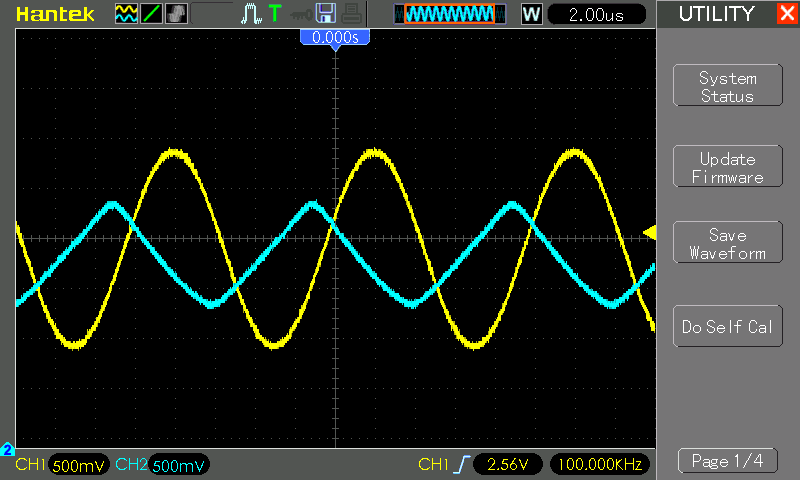Measurements in action…